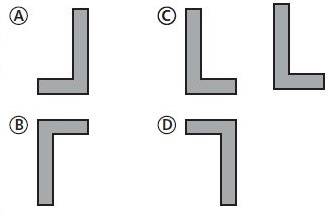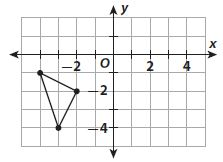Homework Explained - Math Practice 101Dear guest, you are not a registered member. As a guest, you only have read-only access to our books, tests and other practice materials.

As a registered member you can:

Registration is free and doesn't require any type of payment information. Click here to Register.
Go to page:
Chapter 9:Transformations and Congruence; Mixed Review

Selected Response

• Question 1

What would be the orientation of the figure L after a translation of 8 units to the right and 3 units up?• A
• B
• C
• D
• Question 2

Figure A is reflected over the y-axis and then lowered 6 units. Which sequence describes these transformations?

• (x, y) -> (x, -y) and (x, y) -> (x, y - 6)
• (x, y) -> (-x, y) and (x, y) -> (x, y - 6)
• (x, y) -> (x, -y) and (x, y) -> (x - 6, y)
• (x, y) -> (-x, y) and (x, y) -> (x - 6, y)
• Question 3

What quadrant would the triangle be in after a rotation of 90° counterclockwise about the origin?• I
• II
• III
• IV
• Question 4

Which rational number is greater than $$-3 \large\frac{1}{3}$$ but less than $$-\large\frac{4}{5}$$?

• $$-0.4$$
• $$- \large\frac{9}{7}$$
• $$-0.19$$
• $$-\large\frac{22}{5}$$
• Question 5

Which of the following is not true of a trapezoid that has been reflected across the x-axis?

• The new trapezoid is the same size as the original trapezoid.
• The new trapezoid is the same shape as the original trapezoid.
• The new trapezoid is in the same orientation as the original trapezoid.
• The x-coordinates of the new trapezoid are the same as the x-coordinates of the original trapezoid.
• Question 6

A triangle with coordinates (6, 4), (2, −1), and (−3, 5) is translated 4 units left and rotated 180° about the origin. What are the coordinates of its image?

• (2, 4), (-2, -1), (-7, 5)
• (4, 6), (-1, 2), (5, -3)
• (4, -2), (-1, 2), (5, 7)
• (-2, -4), (2, 1), (7, -5)
• Question 7

A rectangle with vertices (3, -2), (3, -4), (7, -2), (7, -4) is reflected across the x-axis and then rotated 90° counterclockwise.

a. In what quadrant does the image lie?

• Question 7

b. What are the vertices of the image?

• Type below:
• Question 7

c. What other transformations produce the same image?

• Type below:

Yes, email page to my online tutor. (if you didn't add a tutor yet, you can add one here)